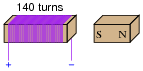# Intermediate Electromagnetism and Electromagnetic Induction

## DC Electric Circuits

• #### Question 1

As an electric current is passed through a coil of wire, it creates a magnetic field. If the magnitude of this current changes over time, so will the strength of the magnetic field.

We also know that a magnetic field flux that changes over time will induce a voltage along the length of a wire coil. Explain how the complementary principles of electromagnetism and electromagnetic induction manifest themselves simultaneously in the same wire coil to produce self-induction.

Also, explain how Lenz’s Law relates to the polarity of the coil’s self-induced voltage.

• #### Question 2

In a simple resistor circuit, the current may be calculated by dividing applied voltage by resistance:Although an analysis of this circuit probably seems trivial to you, I would like to encourage you to look at what is happening here from a fresh perspective. An important principle observed many times in the study of physics is that of equilibrium, where quantities naturally “seek” a state of balance. The balance sought by this simple circuit is equality of voltage: the voltage across the resistor must settle at the same value as the voltage output by the source: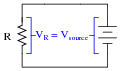If the resistor is viewed as a source of voltage seeking equilibrium with the voltage source, then current must converge at whatever value necessary to generate the necessary balancing voltage across the resistor, according to Ohm’s Law (V = IR). In other words, the resistor’s current achieves whatever magnitude it has to in order to generate a voltage drop equal to the voltage of the source.

This may seem like a strange way of analyzing such a simple circuit, with the resistor “seeking” to generate a voltage drop equal to the source, and current “magically” assuming whatever value it must to achieve that voltage equilibrium, but it is helpful in understanding other types of circuit elements.

For example, here we have a source of DC voltage connected to a large coil of wire through a switch. Assume that the wire coil has negligible resistance (0 Ω):Like the resistor circuit, the coil will “seek” to achieve voltage equilibrium with the voltage source once the switch is closed. However, we know that the voltage induced in a coil is not directly proportional to current as it is with a resistor - instead, a coil’s voltage drop is proportional to the rate of change of magnetic flux over time as described by Faraday’s Law of electromagnetic induction:

 vcoil = N d φ dt

Where,

vcoil = Instantaneous induced voltage, in volts

N = Number of turns in wire coil $$\frac{dφ}{dt}$$ = Instantaneous rate of change of magnetic flux, in webers per second

Assuming a linear relationship between coil current and magnetic flux (i.e. φ doubles when i doubles), describe this simple circuit’s current over time after the switch closes.

• #### Question 3

A very useful method of measuring current through a wire is to measure the strength of the magnetic field around it. This type of ammeter is known as a clamp-on ammeter: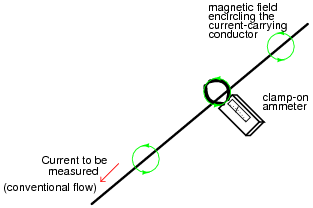Knowing the principle behind this meter’s operation, describe what current values will be indicated by the three clamp-on ammeters in this circuit: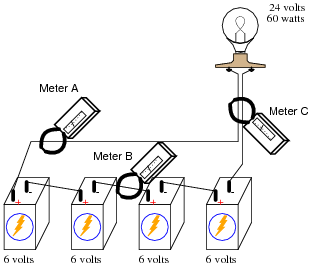Meter A =
Meter B =
Meter C =
• #### Question 4

Write an equation that expresses the amount of magnetic flux (Φ) produced by an electromagnet, given the amount of electric current (I), the number of turns in the wire coil (N), and the reluctance of the core material (ℜ).

• #### Question 5

Plot the relative B-H curves for a sample of air and a sample of iron, in proportion to each other (as much as possible):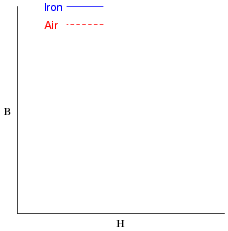What do you notice about the slope (also called the derivative, or $$\frac{dB}{dH}$$ of each plot?

• #### Question 6

If a wire coil with 450 turns is exposed to a magnetic flux increasing at a rate of 0.008 Webers per second, how much voltage will be induced across the coil?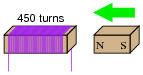• #### Question 7

Lenz’s Law describes the opposition to changes in magnetic flux resulting from electromagnetic induction between a magnetic field and an electrical conductor. One apparatus capable of demonstrating Lenz’s Law is a copper or aluminum disk (electrically conductive, but non-magnetic) positioned close to the end of a powerful permanent magnet. There is no attraction or repulsion between the disk and magnet when there is no motion, but a force will develop between the two objects if either is suddenly moved. This force will be in such a direction that it tries to resist the motion (i.e. the force tries to maintain the gap constant between the two objects):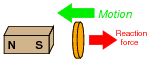We know this force is magnetic in nature. That is, the induced current causes the disk itself to become a magnet in order to react against the permanent magnet’s field and produce the opposing force. For each of the following scenarios, label the disk’s induced magnetic poles (North and South) as it reacts to the motion imposed by an outside force: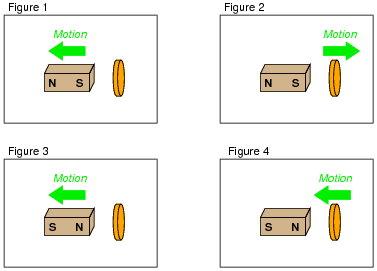• #### Question 8

Combining Lenz’s Law with the right-hand rule (or left-hand rule, if you follow electron flow instead of conventional flow) provides a simple and effective means for determining the direction of induced current in an induction coil. In the following examples, trace the direction of current through the load resistor: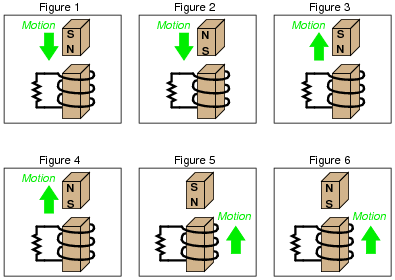• #### Question 9

Write the equation relating electric current and magnetic field strength together in a wire coil. If the current (I) is given in units of amperes, and the number of turns is a simple integer value, what unit of measurement will the magnetic field strength have?

• #### Question 10

What is magnetic saturation?

• #### Question 11

Explain what this graph means, and how it represents both saturation and hysteresis as magnetic phenomena: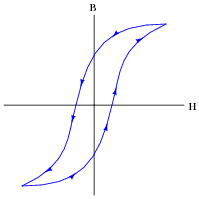• #### Question 12

If an electric current is passed through this wire loop, in which position will it try to orient itself?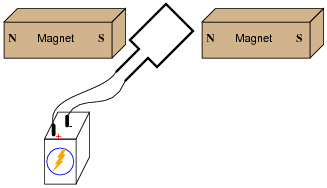If this experiment is carried out, it may be found that the torque generated is quite small without resorting to high currents and/or strong magnetic fields. Devise a way to modify this apparatus so as to generate stronger torques using modest current levels and ordinary magnets.

• #### Question 13

By moving a permanent magnet perpendicularly past a wire, a voltage will be generated between the ends of that wire: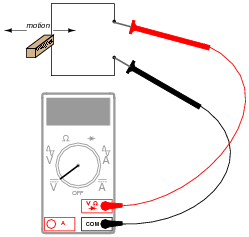Describe what factors determine the polarity and magnitude of this voltage.

• #### Question 14

The relationship between magnetic flux and induced voltage in a wire coil is expressed in this equation, known as Faraday’s Law:

 e = N d φ dt

Where,

e = Instantaneous induced voltage, in volts

N = Number of turns in wire coil

φ = Instantaneous magnetic flux, in webers

t = Time, in seconds

Explain what the mathematical expression $$\frac{d φ}{dt}$$ means, in light of what you know about electromagnetic induction. Hint: the $$\frac{d}{d}$$ notation is borrowed from calculus, and it is called the derivative.

Also, explain why lower-case letters are used (e instead of E, φ instead of Φ) in this equation.

• #### Question 15

How many turns of wire must a coil have in order to induce a voltage of 10.5 volts when exposed to a changing magnetic flux with a rate of 0.0075 Wb/s?

• #### Question 16

If a copper ring is brought closer to the end of a permanent magnet, a repulsive force will develop between the magnet and the ring. This force will cease, however, when the ring stops moving. What is this effect called?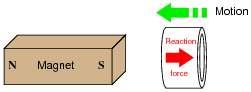Also, describe what will happen if the copper ring is moved away from the end of the permanent magnet.

• #### Question 17

Electromechanical watt-hour meters use an aluminum disk that is spun by an electric motor. To generate a constant “drag” on the disk necessary to limit its rotational speed, a strong magnet is placed in such a way that its lines of magnetic flux pass perpendicularly through the disk’s thickness: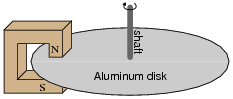Explain the phenomenon behind this magnetic “drag” mechanism, and also explain how the permanent magnet assembly should be re-positioned so that it provides less drag on the disk for the same rotational speed.

• #### Question 18

One context in which to understand Lenz’s Law is the well-known physical law called the Conservation of Energy, which states that energy can neither be created (from nothing) nor destroyed (to nothing). This well-founded law of physics is the general principle forbidding so-called “over-unity” or “free energy” machines, where energy would supposedly be produced from no source whatsoever.

Demonstrate that if Lenz’s Law were reversed, the Conservation of Energy principle would be violated. In other words, imagine what would happen if the effects of Lenz’s Law were exactly opposite in direction, and show how this would result in more energy produced by a system than what is input to that system.

• #### Question 19

Based on your knowledge of Lenz’s Law, explain how one could construct an electromagnetic brake, whereby the energization of an electromagnet coil would produce mechanical “drag” on a rotating shaft without the need for contact between the shaft and a brake pad.

• #### Question 20

Determine the polarity of the coil’s induced voltage for each of the following examples. Be careful to note the direction each coil is wrapped around its core - the coils are not all identical!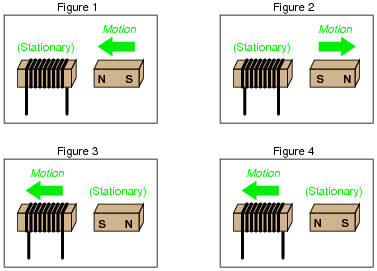• #### Question 21

If a wire coil with 320 turns is exposed to a magnetic flux decreasing at a rate of 0.03 Webers per second (as shown in the illustration), how much voltage will be induced across the coil, and what will its polarity be?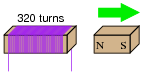• #### Question 22

If a wire coil with 1100 turns is exposed to a magnetic flux increasing at a rate of 0.07 Webers per second (as shown in the illustration), how much voltage will be induced across the coil, and what will its polarity be?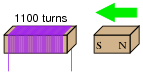• #### Question 23

Calculate the necessary magnetic flux rate-of-change over time (in units of Webers per second) as well as the direction of magnet motion (either toward or away from the coil) to induce a voltage of 13.5 volts in the polarity shown: